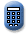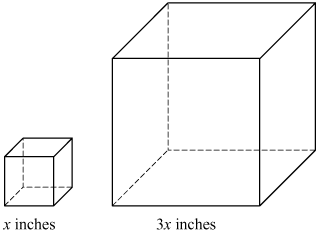Massachusetts Comprehensive Assessment SystemReporting Category: Geometry
Standard: 10.M.3 - Relate changes in the measurement of one attribute of an object to changes in other attributes, e.g., how changing the radius or height of a cylinder affects its surface area or volume.
Standard: CCSS.Math.Content.7.G.B.6 - Solve real-world and mathematical problems involving area, volume and surface area of two- and three-dimensional objects composed of triangles, quadrilaterals, polygons, cubes, and right prisms.

A small cube and a large cube with edge lengths represented by expressions are shown in the diagram below.The small cube has a volume of 64 cubic inches.

1. What is the value of x? Show or explain how you got your answer.
2. What is the surface area, in square inches, of the small cube? Show or explain how you got your answer.
3. The surface area of the large cube is how many times the surface area of the small cube? Show or explain how you got your answer.
4. The volume of the large cube is how many times the volume of the small cube? Show or explain how you got your answer.

## Scoring Guide and Sample Student Work

Select a score point in the table below to view the sample student response.

ScoreDescription
4 The student response demonstrates an exemplary understanding of the Geometry concepts involved in solving mathematical problems involving volume and surface area of three-dimensional objects. The student compares measurements of different-sized cubes.
4
3 The student response demonstrates a good understanding of the Geometry concepts involved in solving mathematical problems involving volume and surface area of three-dimensional objects. Although there is significant evidence that the student was able to recognize and apply the concepts involved, some aspect of the response is flawed. As a result the response merits 3 points.
2 The student response demonstrates a fair understanding of the Geometry concepts involved in solving mathematical problems involving volume and surface area of three-dimensional objects. While some aspects of the task are completed correctly, others are not. The mixed evidence provided by the student merits 2 points.
1 The student response demonstrates a minimal understanding of the Geometry concepts involved in solving mathematical problems involving volume and surface area of three-dimensional objects.
0 The student response contains insufficient evidence of an understanding of the Geometry concepts involved in solving mathematical problems involving volume and surface area of three-dimensional objects to merit any points.
Note: There are 2 sample student responses for Score Point 4.

Question 17: Number and Quantity
Question 20: Algebra and Functions
Question 21: Algebra and Functions
Question 36: Geometry
Question 41: Statistics and Probability
Question 42: Geometry

Last Updated: July 1, 2019